Courses

# Statistical Description of Data CA CPT Notes | EduRev

## CA CPT : Statistical Description of Data CA CPT Notes | EduRev

The document Statistical Description of Data CA CPT Notes | EduRev is a part of the CA CPT Course Business Mathematics and Logical Reasoning & Statistics.
All you need of CA CPT at this link: CA CPT

INTRODUCTION OF STATISTICS
The modern development in the field of not only Management, Commerce, Economics, Social Sciences, Mathematics and so on but also in our life like public services, defence, banking, insurance sector, tourism and hospitality, police and military etc. are dependent on a particular subject known as statistics. Statistics does play a vital role in enriching a specific domain by collecting data in that field, analysing the data by applying various statistical techniques and finally making statistical inferences about the domain. In the present world, statistics has almost a universal application. Our Government applies statistics to make the economic planning in an effective and a pragmatic way. The businessman plan and expand their horizons of business on the basis of the analysis of the feedback data. The political parties try to impress the general public by presenting the statistics of their performances and accomplishments. Most of the research scholars of today also apply statistics to present their research papers in an authoritative manner. Thus the list of people using statistics goes on and on and on. Due to these factors, it is necessary to study the subject of statistics in an objective manner.
History of Statistics
Going through the history of ancient period and also that of medieval period, we do find the mention of statistics in many countries. However, there remains a question mark about the origin of the word ‘statistics’. One view is that statistics is originated from the Latin word ‘status’. According to another school of thought, it had its origin in the Italian word ‘statista’. Some scholars believe that the German word ‘statistik’ was later changed to statistics and another suggestion is that the French word ‘statistique’ was made as statistics with the passage of time.In those days, statistics was analogous to state or, to be more precise, the data that are collected and maintained for the welfare of the people belonging to the state. We are thankful to Kautilya who had kept a record of births and deaths as well as some other precious records in his famous book ‘Arthashastra’ during Chandragupta’s reign in the fourth century B.C. During the reign of Akbar in the sixteenth century A.D. We find statistical records on agriculture in Ain-i-Akbari written by Abu Fazl. Referring to Egypt, the first census was conducted by the Pharaoh during 300 B.C. to 2000 B.C.
Definition of Statistics
We may define statistics either in a singular sense or in a plural sense Statistics, when used as a plural noun, may be defined as data qualitative as well as quantitative, that are collected, usually with a view of having statistical analysis. However, statistics, when used as a singular noun, may be defined, as the scientific method that is employed for collecting, analysing and presenting data, leading finally to drawing statistical inferences about some important characteristics it means it is ‘science of counting’ or ‘science of averages’.
Application of statistics
Among various applications of statistics, let us confine our discussions to the fields of Economics, Business Management and Commerce and Industry.
Economics
Modern developments in Economics have the roots in statistics. In fact, Economics and Statistics are closely associated. Time Series Analysis, Index Numbers, Demand Analysis etc. are some overlapping areas of Economics and Statistics. In this connection, we may also mention Econometrics – a branch of Economics that interact with statistics in a very positive way. Conducting socio-economic surveys and analysing the data derived from it are made with the help of different statistical methods. Regression analysis, one of the numerous applications of statistics, plays a key role in Economics for making future projection of demand of goods, sales, prices, quantities etc. which are all ingredients of Economic planning.
Gone are the days when the managers used to make decisions on the basis of hunches, intuition or trials and errors. Now a days, because of the never-ending complexity in the business and industry environment, most of the decision making processes rely upon different quantitative techniques which could be described as a combination of statistical methods and operations research techniques. So far as statistics is concerned, inferences about the universe from the knowledge of a part of it, known as sample, plays an important role in the development of certain criteria. Statistical decision theory is another component of statistics that focuses on the analysis of complicated business strategies with a list of alternatives – their merits as well as demerits.
Statistics in Commerce and Industry
In this age of cut-throat competition, like the modern managers, the industrialists and the businessmen are expanding their horizons of industries and businesses with the help of statistical procedures. Data on previous sales, raw materials, wages and salaries, products of identical nature of other factories etc are collected, analysed and experts are consulted in order to maximise profits. Measures of central tendency and dispersion, correlation and regression analysis, time series analysis, index numbers, sampling, statistical quality control are some of the statistical methods employed in commerce and industry.
Limitations of Statistics
Before applying statistical methods, we must be aware of the following limitations:
I Statistics deals with the aggregates. An individual, to a statistician has no significance except the fact that it is a part of the aggregate.
II Statistics is concerned with quantitative data. However, qualitative data also can be converted to quantitative data by providing a numerical description to the corresponding qualitative data.
III Future projections of sales, production, price and quantity etc. are possible under a specific set of conditions. If any of these conditions is violated, projections are likely to be inaccurate.
IV The theory of statistical inferences is built upon random sampling. If the rules for random sampling are not strictly adhered to, the conclusion drawn on the basis of these unrepresentative samples would be erroneous. In other words, the experts should be consulted before deciding the sampling scheme.
COLLECTION OF DATA
We may define ‘data’ as quantitative information about some particular characteristic(s) under consideration. Although a distinction can be made between a qualitative characteristic and a quantitative characteristic but so far as the statistical analysis of the characteristic is concerned, we need to convert qualitative information to quantitative information by providing a numeric descriptions to the given characteristic. In this connection, we may note that a quantitative characteristic is known as a variable or in other words, a variable is a measurable quantity. Again, a variable may be either discrete or continuous. When a variable assumes a finite or a countably infinite number of isolated values, it is known as a discrete variable. Examples of discrete variables may be found in the number of petals in a flower, the number of misprints a book contains, the number of road accidents in a particular locality and so on. A variable, on the other hand, is known to be continuous if it can assume any value from a given interval. Examples of continuous variables may be provided by height, weight, sale, profit and so on. Finally, a qualitative characteristic is known as an attribute. The gender of a baby, the nationality of a person, the colour of a flower etc. are examples of attributes.
We can broadly classify data as
(a) Primary;
(b) Secondary.
Collection of data plays the very important role for any statistical analysis. The data which are collected for the first time by an investigator or agency are known as primary data whereas the data are known to be secondary if the data, as being already collected, are used by a different person or agency. Thus, if Prof. Das collects the data on the height of every student in his class, then these would be primary data for him. If, however, another person, say, Professor Bhargava uses the data, as collected by Prof. Das, for finding the average height of the students belonging to that class, then the data would be secondary for Prof. Bhargava.
Collection of Primary Data
The following methods are employed for the collection of primary data:
(i) Interview method;
(ii) Mailed questionnaire method;
(iii) Observation method;
(iv) Questionnaries filled and sent by enumerators.
Interview method again could be divided into (a) Personal Interview method, (b) Indirect Interview method and (c) Telephone Interview method.
In personal interview method, the investigator meets the respondents directly and collects the required information then and there from them. In case of a natural calamity like a super cyclone or an earthquake or an epidemic like plague, we may collect the necessary data much more quickly and accurately by applying this method.
If there are some practical problems in reaching the respondents directly, as in the case of a rail accident, then we may take recourse for conducting Indirect Interview where the investigator collects the necessary information from the persons associated with the problems.
Telephone interview method is a quick and rather non-expensive way to collect the primary data where the relevant information can be gathered by the researcher himself by contacting the interviewee over the phone. The first two methods, though more accurate, are inapplicable for covering a large area whereas the telephone interview, though less consistent, has a wide coverage.
The amount of non-responses is maximum for this third method of data collection.
Mailed questionnaire method comprises of framing a well-drafted and soundly-sequenced questionnaire covering all the important aspects of the problem under consideration and sending them to the respondents with pre-paid stamp after providing all the necessary guidelines for filling up the questionnaire. Although a wide area can be covered using the mailed questionnaire method, the amount of non-responses is likely to be maximum in this method.
In observation method, data are collected, as in the case of obtaining the data on the height and weight of a group of students, by direct observation or using instrument. Although this is likely to be the best method for data collection, it is time consuming, laborious and covers only a small area. Questionnaire form of data collection is used for larger enquiries from the persons who are surveyed. Enumerators collects information directly by interviewing the persons having information : Question are explained and hence data is collected.
Sources of Secondary Data
There are many sources of getting secondary data. Some important sources are listed below:
(a) International sources like WHO, ILO, IMF, World Bank etc.
(b) Government sources like Statistical Abstract by CSO, Indian Agricultural Statistics by the Ministry of Food and Agriculture and so on.
(c) Private and quasi-government sources like ISI, ICAR, NCERT etc.
(d) Unpublished sources of various research institutes, researchers etc.
Scrutiny of Data
Since the statistical analyses are made only on the basis of data, it is necessary to check whether the data under consideration are accurate as well as consistence. No hard and fast rules can be recommended for the scrutiny of data. One must apply his intelligence, patience and experience while scrutinising the given information.
Errors in data may creep in while writing or copying the answer on the part of the enumerator. A keen observer can easily detect that type of error. Again, there may be two or more series of figures which are in some way or other related to each other. If the data for all the series are provided, they may be checked for internal consistency. As an example, if the data for population, area and density for some places are given, then we may verify whether they are internally consistent by examining whether the relationA good statistician can also detect whether the returns submitted by some enumerators are exactly of the same type thereby implying the lack of seriousness on the part of the enumerators. The bias of the enumerator also may be reflected by the returns submitted by him. This type of error can be rectified by asking the enumerator(s) to collect the data for the disputed cases once again.
PRESENTATION OF DATA
Once the data are collected and verified for their homogeneity and consistency, we need to
present them in a neat and condensed form highlighting the essential features of the data. Any statistical analysis is dependent on a proper presentation of the data under|consideration.
Classification or Organisation of Data
It may be defined as the process of arranging data on the basis of the characteristic under consideration into a number of groups or classes according to the similarities of the observations. Following are the objectives of classification of data:
(a) It puts the data in a neat, precise and condensed form so that it is easily understood and interpreted.
(b) It makes comparison possible between various characteristics, if necessary, and thereby finding the association or the lack of it between them.
(c) Statistical analysis is possible only for the classified data.

(d) It eliminates unnecessary details and makes data more readily understandable. Data may be classified as -
(i) Chronological or Temporal or Time Series Data;
(ii) Geographical or Spatial Series Data;
(iii) Qualitative or Ordinal Data;
(iv) Quantitative or Cardinal Data.
When the data are classified in respect of successive time points or intervals, they are known as time series data. The number of students appeared for CA final for the last twenty years, the production of a factory per month from 2000 to 2015 etc. are examples of time series data.
Data arranged region wise are known as geographical data. If we arrange the students appeared for CA final in the year 2015 in accordance with different states, then we come across Geographical Data.
Data classified in respect of an attribute are referred to as qualitative data. Data on nationality, gender, smoking habit of a group of individuals are examples of qualitative data. Lastly, when the data are classified in respect of a variable, say height, weight, profits, salaries etc., they are known as quantitative data.
Data may be further classified as frequency data and non-frequency data. The qualitative as well as quantitative data belong to the frequency group whereas time series data and geographical data belong to the non-frequency group.
Mode of Presentation of Data
Next, we consider the following mode of presentation of data:
(a) Textual presentation;
(b) Tabular presentation or Tabulation;
(c) Diagrammatic representation.

(a) Textual presentation
This method comprises presenting data with the help of a paragraph or a number of
paragraphs. The official report of an enquiry commission is usually made by textual
presentation. Following is an example of textual presentation.
‘In 2009, out of a total of five thousand workers of Roy Enamel Factory, four thousand

and two hundred were members of a Trade Union. The number of female workers was

twenty per cent of the total workers out of which thirty per cent were members of the

In 2010, the number of workers belonging to the trade union was increased by twenty per

cent as compared to 2009 of which four thousand and two hundred were male. The

number of workers not belonging to trade union was nine hundred and fifty of which

four hundred and fifty were females.’

The merit of this mode of presentation lies in its simplicity and even a layman can present

data by this method. The observations with exact magnitude can be presented with the

help of textual presentation. Furthermore, this type of presentation can be taken as the

first step towards the other methods of presentation.

Textual presentation, however, is not preferred by a statistician simply because, it is dull,

monotonous and comparison between different observations is not possible in this method.

For manifold classification, this method cannot be recommended.
(b) Tabular presentation or Tabulation
Tabulation may be defined as systematic presentation of data with the help of a statistical table having a number of rows and columns and complete with reference number, title, description of rows as well as columns and foot notes, if any.
We may consider the following guidelines for tabulation :
I A statistical table should be allotted a serial number along with a self-explanatory title.
II The table under consideration should be divided into caption, Box-head, Stub and Body. Caption is the upper part of the table, describing the columns and sub-columns, if any. The Box-head is the entire upper part of the table which includes columns and sub-column numbers, unit(s) of measurement along with caption. Stub is the left part of the table providing the description of the rows. The body is the main part of the table that contains the numerical figures.
III The table should be well-balanced in length and breadth.
IV The data must be arranged in a table in such a way that comparison(s) between different figures are made possible without much labour and time. Also the row totals, column totals, the units of measurement must be shown.
V The data should be arranged intelligently in a well-balanced sequence and the presentation of data in the table should be appealing to the eyes as far as practicable.
VI Notes describing the source of the data and bringing clarity and, if necessary, about any rows or columns known as footnotes, should be shown at the bottom part of the table.
The textual presentation of data, relating to the workers of Roy Enamel Factory is shown in the following table.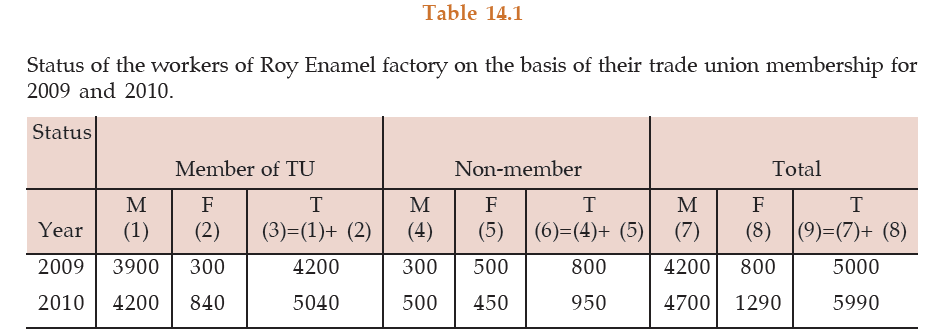Source:

Footnote: TU, M, F and T stand for trade union, male, female and total respectively.

The tabulation method is usually preferred to textual presentation as

(i) It facilitates comparison between rows and columns.

(ii) Complicated data can also be represented using tabulation.

(iii) It is a must for diagrammatic representation.

(iv) Without tabulation, statistical analysis of data is not possible.
(c) Diagrammatic representation of data
Another alternative and attractive representation of statistical data is provided by charts,

diagrams and pictures. Unlike the first two methods of representation of data, diagrammatic representation can be used for both the educated section and uneducated section of the society. Furthermore, any hidden trend present in the given data can be noticed only in this mode of representation. However, compared to tabulation, this is less accurate. So if there is a priority for accuracy, we have to recommend tabulation.
We are going to consider the following types of diagrams :
I Line diagram or Historiagram;
II Bar diagram;

III Pie chart.
I Line diagram or Historiagram

When the data vary over time, we take recourse to line diagram. In a simple line diagram,

we plot each pair of values of (t, yt), yt representing the time series at the time point t in the t–yt plane. The plotted points are then joined successively by line segments and the resulting chart is known as line-diagram.
When the time series exhibit a wide range of fluctuations, we may think of logarithmic or

ratio chart where Log yt and not yt is plotted against t. We use Multiple line chart for

representing two or more related time series data expressed in the same unit and multiple

– axis chart in somewhat similar situations if the variables are expressed in different units.
II Bar diagram
There are two types of bar diagrams namely, Horizontal Bar diagram and Vertical Bar diagram. While horizontal bar diagram is used for qualitative data or data varying over space, the vertical bar diagram is associated with quantitative data or time series data. Bars i.e. rectangles of equal width and usually of varying lengths are drawn either horizontally or vertically. We consider Multiple or Grouped Bar diagrams to compare related series. Component or sub-divided Bar diagrams are applied for representing data divided into a number of components. Finally, we use Divided Bar charts or Percentage Bar diagrams for comparing different components of a variable and also the relating of the components to the whole. For this situation, we may also use Pie chart or Pie diagram or circle diagram.
ILLUSTRATIONS:
Example: The profits in lakhs of Rupees of an industrial house for 2009, 2010, 2011, 2012, 2013, 2014, and 2015 are 5, 8, 9, 6, 12, 15 and 24 respectively. Represent these data using a suitable diagram.
SOLUTION:
We can represent the profits for 7 consecutive years by drawing either a line chart or a vertical bar chart shows a line chart and figure shows the corresponding vertical bar chart.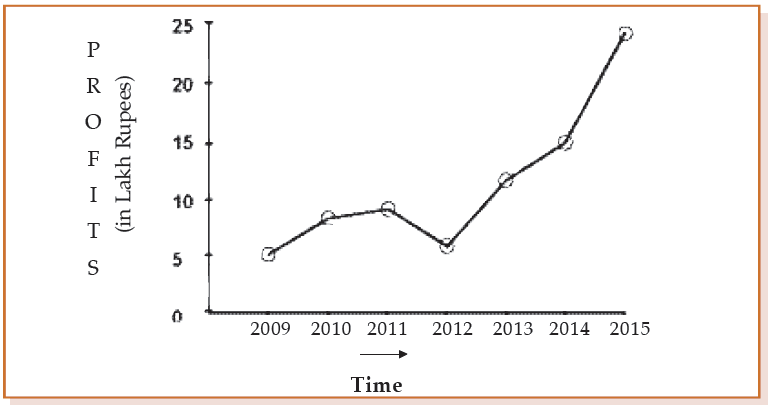Showing line chart for the Profit of an Industrial House during 2002 to 2008.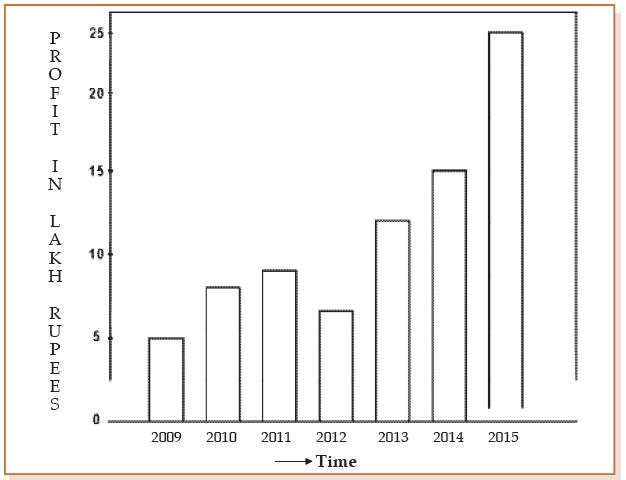Showing vertical bar diagram for the Profit of an Industrial house from 2007 to 2015.
Example: The production of wheat and rice of a region are given below :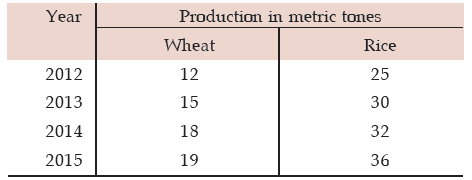Represent this information using a suitable diagram.
Solution:
We can represent this information by drawing a multiple line chart. Alternately, a multiple bar diagram may be considered. These are depicted in figure 14.3 and 14.4 respectively.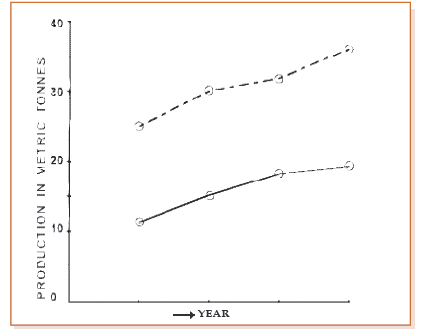Multiple line chart showing production of wheat and rice of a region during 2012–2015.

(Dotted line represent production of rice and continuous line that of wheat).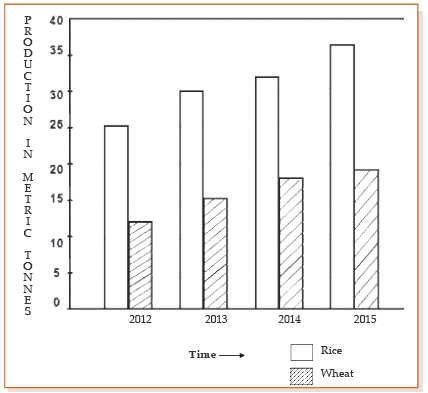Multiple bar chart representing production of rice and wheat from 2012 to 2015.
Example: Draw an appropriate diagram with a view to represent the following data :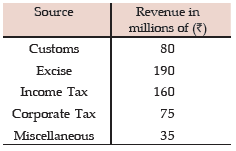Solution:
Pie chart or divided bar chart would be the ideal diagram to represent this data. We consider Pie chart.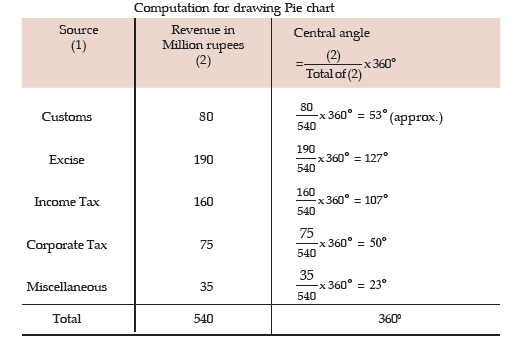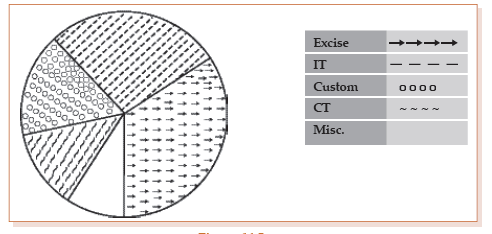Pie chart showing the distribution of Revenue

FREQUENCY DISTRIBUTION
As discussed in the previous section, frequency data occur when we classify statistical data in respect of either a variable or an attribute. A frequency distribution may be defined as a tabular representation of statistical data, usually in an ascending order, relating to a measurable characteristic according to individual value or a group of values of the characteristic under study.
In case, the characteristic under consideration is an attribute, say nationality, then the tabulation is made by allotting numerical figures to the different classes the attribute may belong like, in this illustration, counting the number of Indian, British, French, German and so on. The qualitative characteristic is divided into a number of categories or classes which are mutually exclusive and exhaustive and the figures against all these classes are recorded. The figure corresponding to a particular class, signifying the number of times or how frequently a particular class occurs is known as the frequency of that class. Thus, the number of Indians, as found from the given data, signifies the frequency of the Indians. So frequency distribution is a statistical table that distributes the total frequency to a number of classes.
When tabulation is done in respect of a discrete random variable, it is known as Discrete or Ungrouped or simple Frequency Distribution and in case the characteristic under consideration is a continuous variable, such a classification is termed as Grouped Frequency Distribution. In case of a grouped frequency distribution, tabulation is done not against a single value as in the case of an attribute or a discrete random variable but against a group of values. The distribution of the number of car accidents in Delhi during 12 months of the year 2005 is an example of a ungrouped frequency distribution and the distribution of heights of the students of St. Xavier’s College for the year 2004 is an example of a grouped frequency distribution.
Example :
Following are the records of babies born in a nursing home in Bangalore during
a week (B denoting Boy and G for Girl) :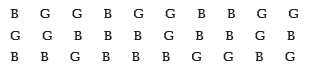Construct a frequency distribution according to gender.
Solution:

In order to construct a frequency distribution of babies in accordance with their gender, we count the number of male births and that of female births and present this information in the following table.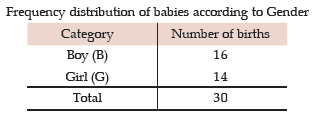Frequency Distribution of a Variable
For the construction of a frequency distribution of a variable, we need to go through the following steps :
I Find the largest and smallest observations and obtain the difference between them, known as Range, in case of a continuous variable.
II Form a number of classes depending on the number of isolated values assumed by a discrete variable. In case of a continuous variable, find the number of class intervals using the relation, No. of class Interval X class length≅Range.
III Present the class or class interval in a table known as frequency distribution table.
IV Apply ‘tally mark’ i.e. a stroke against the occurrence of a particulars value in a class or class interval.
V Count the tally marks and present these numbers in the next column, known as frequency column, and finally check whether the total of all these class frequencies tally with the total number of observations.
Example : A review of the first 30 pages of a statistics book reveals the following printing

mistakes: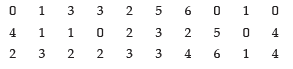Make a frequency distribution of printing mistakes.
Solution:
Since x, the printing mistakes, is a discrete variable, x can assume seven values 0, 1, 2, 3, 4, 5 and 6. Thus we have 7 classes, each class comprising a single value.
Frequency Distribution of the number of printing mistakes of the first 30 pages of a book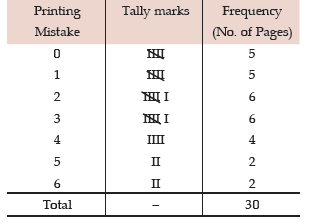Example : Following are the weights in kgs. of 36 BBA students of St. Xavier’s College.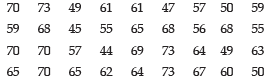Construct a frequency distribution of weights, taking class length as 5.
Solution: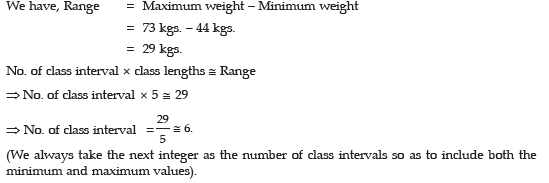Frequency Distribution of weights of 36 BBA Students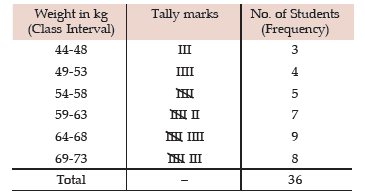Some important terms associated with a frequency distribution
Class Limit (CL)
Corresponding to a class interval, the class limits may be defined as the minimum value and the maximum value the class interval may contain. The minimum value is known as the lower class limit (LCL) and the maximum value is known as the upper class limit (UCL). For the frequency distribution of weights of BBA Students, the LCL and UCL of the first class interval are 44 kgs. and 48 kgs. respectively.
Class Boundary (CB)
Class boundaries may be defined as the actual class limit of a class interval. For overlapping classification or mutually exclusive classification that excludes the upper class limits like 10–20, 20–30, 30–40, ……… etc. the class boundaries coincide with the class limits. This is usually done for a continuous variable. However, for non-overlapping or mutually inclusive classification that includes both the class limits like 0–9, 10–19, 20–29,…… which is usually applicable for a discrete variable, we have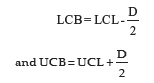where D is the difference between the LCL of the next class interval and the UCL of the given class interval. For the data presented in table 10.5, LCB of the first class interval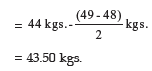and the corresponding UCB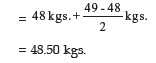Mid-point or Mid-value or class mark

Corresponding to a class interval, this may be defined as the total of the two class limits or class boundaries to be divided by 2. Thus, we have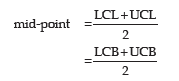Referring to the distribution of weight of BBA students, the mid-points for the first two class intervals are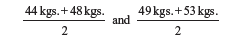i.e. 46 kgs. and 51 kgs. respectively.
Width or size of a class interval

The width of a class interval may be defined as the difference between the UCB and the LCB of that class interval. For the distribution of weights of BBA students, C, the class length or width is 48.50 kgs. – 43.50 kgs. = 5 kgs. for the first class interval. For the other class intervals also, C remains same.
Cumulative Frequency

The cumulative frequency corresponding to a value for a discrete variable and corresponding to a class boundary for a continuous variable may be defined as the number of observations less than the value or less than or equal to the class boundary. This definition refers to the less than cumulative frequency. We can define more than cumulative frequency in a similar manner. Both types of cumulative frequencies are shown in the following table.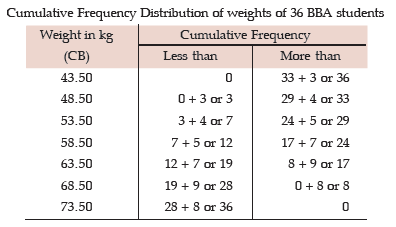Frequency density of a class interval
It may be defined as the ratio of the frequency of that class interval to the corresponding class length. The frequency densities for the first two class intervals of the frequency distribution of weights of BBA students are 3/5 and 4/5 i.e. 0.60 and 0.80 respectively. Relative frequency and percentage frequency of a class interval
Relative frequency of a class interval may be defined as the ratio of the class frequency to the total frequency. Percentage frequency of a class interval may be defined as the ratio of class frequency to the total frequency, expressed as a percentage. For the last example, the relative frequencies for the first two class intervals are 3/36 and 4/36 respectively and the percentage frequencies are 300/36 and 400/36 respectively. It is quite obvious that whereas the relative frequencies add up to unity, the percentage frequencies add up to one hundred.
GRAPHICAL REPRESENTATION OF A FREQUENCY

DISTRIBUTION
We consider the following types of graphical representation of frequency distribution :

(i) Histogram or Area diagram;

(ii) Frequency Polygon;

(iii) Ogives or cumulative Frequency graphs.

(i) Histogram or Area diagram

This is a very convenient way to represent a frequency distribution. Histogram helps us to

get an idea of the frequency curve of the variable under study. Some statistical measure

can be obtained using a histogram. A comparison among the frequencies for different

class intervals is possible in this mode of diagrammatic representation.

In order to draw a histogram, the class limits are first converted to the corresponding class

boundaries and a series of adjacent rectangles, one against each class interval, with the

class interval as base or breadth and the frequency or frequency density usually when the

class intervals are not uniform as length or altitude, is erected. The histogram for the

distribution of weight of 36 BBA students is shown below. The mode of the weights has

also been determined using the histogram.
i.e. Mode = 66.50 kgs.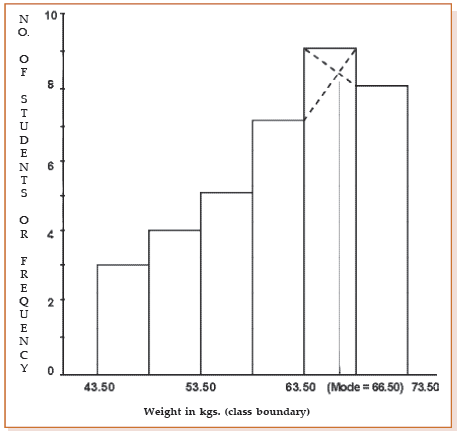Showing histogram for the distribution of weight of 36 BBA students
(ii) Frequency Polygon
Usually frequency polygon is meant for single frequency distribution. However, we also apply it for grouped frequency distribution provided the width of the class intervals remains the same. A frequency curve can be regarded as a limiting form of frequency polygon. In order to draw a frequency polygon, we plot (xi, fi) for i = 1, 2, 3, ……….. n with xi denoting the mid-point of the its class interval and fi, the corresponding frequency, n being the number of class intervals. The plotted points are joined successively by line segments and the figure, so drawn, is given the shape of a polygon, a closed figure, by joining the two extreme ends of the drawn figure to two additional points (x0,0) and (xn+1,0).
The frequency polygon for the distribution of weights of BBA students is shown in Figure 14.7. We can also obtain a frequency polygon starting with a histogram by adding the mid-points of the upper sides of the rectangles successively and then completing the figure by joining the two ends as before.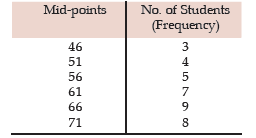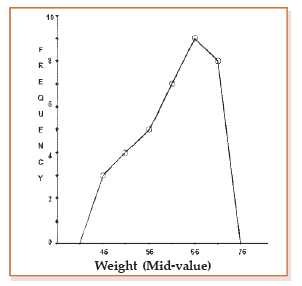Showing frequency polygon for the distribution of height of 36 BBA students
(iii) Ogives or Cumulative Frequency Graph

By plotting cumulative frequency against the respective class boundary, we get ogives. As

such there are two ogives – less than type ogives, obtained by taking less than cumulative

frequency on the vertical axis and more than type ogives by plotting more than type

cumulative frequency on the vertical axis and thereafter joining the plotted points

successively by line segments. Ogives may be considered for obtaining quartiles graphically. If a perpendicular is drawn from the point of intersection of the two ogives on the horizontal axis, then the x-value of this point gives us the value of median, the second or middle quartile. Ogives further can be put into use for making short term projections.

Figure 14.8 depicts the ogives and the determination of the quartiles. This figure give us

the following information.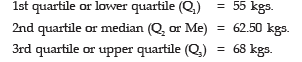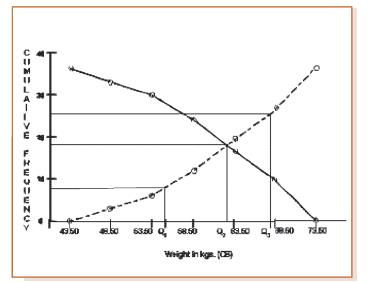Showing the ogives for the distribution of weights of 36 BBA students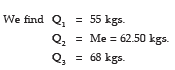A frequency curve is a smooth curve for which the total area is taken to be unity. It is a limiting form of a histogram or frequency polygon. The frequency curve for a distribution can be obtained by drawing a smooth and free hand curve through the mid-points of the upper sides of the rectangles forming the histogram.
There exist four types of frequency curves namely

(a) Bell-shaped curve;

(b) U-shaped curve;

(c) J-shaped curve;

(d) Mixed curve.
Most of the commonly used distributions provide bell-shaped curve, which, as suggested by the name, looks almost like a bell. The distribution of height, weight, mark, profit etc. usually belong to this category. On a bell-shaped curve, the frequency, starting from a rather low value, gradually reaches the maximum value, somewhere near the central part and then gradually decreases to reach its lowest value at the other extremity.
For a U-shaped curve, the frequency is minimum near the central part and the frequency slowly but steadily reaches its maximum at the two extremities. The distribution of Kolkata bound commuters belongs to this type of curve as there are maximum number of commuters during the peak hours in the morning and in the evening.
The J-shaped curve starts with a minimum frequency and then gradually reaches its maximum frequency at the other extremity. The distribution of commuters coming to Kolkata from the early morning hour to peak morning hour follows such a distribution. Sometimes, we may also come across an inverted J-shaped frequency curve.
Lastly, we may have a combination of these frequency curves, known as mixed curve. These are exhibited in the following figures.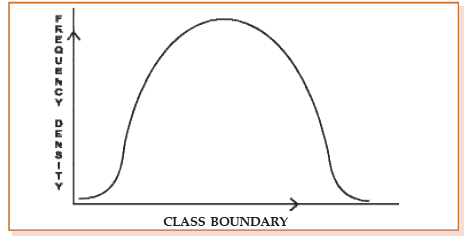Bell-shaped curve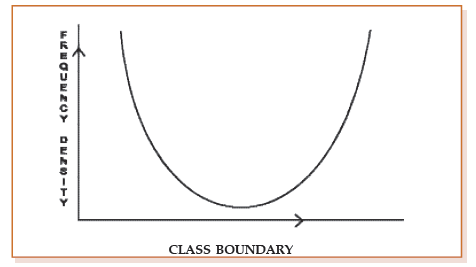U-shaped curve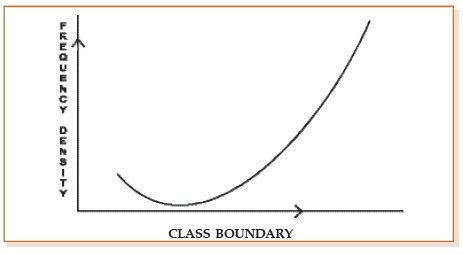J-shaped curve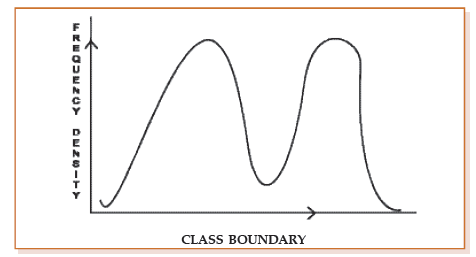Mixed curve

Offer running on EduRev: Apply code STAYHOME200 to get INR 200 off on our premium plan EduRev Infinity!

## Business Mathematics and Logical Reasoning & Statistics

33 videos|101 docs|87 tests

,

,

,

,

,

,

,

,

,

,

,

,

,

,

,

,

,

,

,

,

,

;